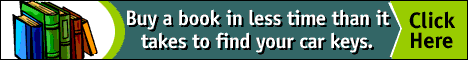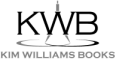Abstract. Martin Euser researches the factor root-(2N - 1) and its interesting relations between musical proportions and Pythagorean triangles. The simple scheme N +/- root-N is also interesting as a generative set of pairs of numbers. This set looks like a prototype for the generative set of pairs of numbers discussed in a previous article by the author. The findings are presented summarily and it is left to the reader to elaborate upon them.

#Pythagorean Triangles and Musical Proportions

Martin Euser
Bosweg 3
3922 GJ Elst (Utrecht)
The Netherlands

INTRODUCTION
S
ince writing a previous article on Sacred Geometry I have done a little research on the factor root-(2N - 1) and found some interesting relations between musical proportions and Pythagorean triangles. Furthermore, I have looked into the matter of the 'root-factor' scheme a bit further and found that the simple scheme N +/- root-N is also interesting as a generative set of pairs of numbers. This set looks like a prototype for the generative set of pairs of numbers I have discussed in a previous article. I will present these findings summarily and leave it to the reader to elaborate upon them.

ROOT-(2N-1) AS A GEOMETRIC MEAN
T
he thing that got me started this time was a reference to Adrastus by Theon of Smyrna. Adrastus is said to have said that out of all the different mathematical means the geometrical mean is the most important. Why this is so, is not made clear.

However it may be, I focused on the factor root-(2N-1) and thought it would be interesting to consider this factor as a geometrical mean. Hence I sought two terms that would yield this factor. Proceed as follows:

a : root-(2N-1) = root-(2N-1): b

hence: ab = 2N - 1.

I dissolved 2N-1 into two component factors:

root-(2N) + 1

and

root-(2N) -1,

which can be taken to be a respectively b.

Some interesting features arise from these factors: a - b = 2 for all N, and root-(2N) yields integral values for N = 2 c2, namely: 2c.

When you elaborate these values for 2c you will find 2,4,6,8,10, etc., which is a series that shows musical proportions (4:2 is an octave, 6:4 is a fifth, etc.).

The number 2 is prevalent here. The terms a and b form interesting series when N=2c2, namely:

for a: 3,5,7,9, ..

for c: 1,3,5,7,..

which are of the form 2N+1 or 2N -1 (N >= 1).

One hypothesis could be that these values are the input in the term root-(2N-1) in the root-scheme formula. This would link Pythagorean triangles with this formula (see below why this could be so).

PROPORTIONS
Since proportions are the thing we're after in Sacred Geometry, I had a look at the proportions of root-(p/q) where p = 2d2 and q = 2e2.

This formula gives rise to musical proportions:

for d = 2 and e = 1 it yields: 2/1 = 2 , the octave

for d = 3 and e = 2 it yields: 3/2 , the third or fifth

for d = 4 and e = 3 it yields: 4/3, the fourth

etc.

The formula 2N2 is thus seen to be connected with musical proportions.

Now, for some intuitive reason, I started to combine the variables d and e in the following fashion:

add squares of d and e: d2 + e2 = r;

subtract squares of d and e: d2 - e2 = p;

double product of d and e : 2de = q.

for d=2 and e=1 this procedure renders:

r = d2 + e2 = 5 ; p= d2 - e2 =3 ; q = 2de = 4,

which reminded me of the famous Pythagorean triangle with sides 3, 4 and 5.

This triangle is common in the physical shapes/forms of many fishes, as Doczi shows in his book on the power of limits.

For d=3 and e=2 we get:

q = 2de = 12 ; r = d2 + e2 = 13 ; p = d2 - e2 =5,

and, yes, this is another famous Pythagorean triangle with sides 5, 12, 13. And so on for higher values for d and e.

So, a correlation is established between Pythagorean triangles and musical proportions, as I have proven here mathematically. These triangles seem to be connected to the root-number scheme too. I would not be surprised when they would play a vital role in the structure-function-order of nature (e.g. in atomic shell structure). After all, Pythagoras was a very wise man, who taught the mysteries in his Mystery-school and it is very likely that he knew a lot of the workings of nature, being a (high degree) initiate himself.

Very simple properties for the Pythagorean triangles can be derived from what I have deduced already:

r - q = d2 + e2 - 2de = (d - e)2 = 1

because e is taken to be d + 1.

r - p = d2 + e2 - ( e2 - d2) = 2d2,

which is the famous formula for periodicity of electronic shell-structure.
Interestingly enough, r + p = 2e2, which refers to the same formula, but to the next shell-number (e = d + 1).

This means that the following relations between p, q, r and d, e hold:

p = d + e = 2(e - 1) ; q = 2de = 2(e2 -e) ;
r = q + 1 = 2de + 1 = 2(e2 - e) + 1

(Figure 1).

Thus, the Pythagorean triangles can be characterized by one variable, e, which starts with value e = 2 to render the 3,4,5 triangle and so on, proceeding in incremental fashion e = 3, e = 4, etc.
Replace e by N, and we get p = 2N -1, the term appearing in the root-(2N-1).

Now, q = 2N(N-1), which equals the double of the geometric mean of the pair,

N2 + N [root-(2N-1)]

and

N2 - N [root-(2N-1)].

This is an interesting result, the ramifications of which are not yet clear.

There may be a relation to a very simple generative set of number-pairs:

N + root-N

and

N - root-N.

These pairs have the following means:

A= N ; G = root-[N*(N-1)]; H = N -1,

which means are connected to the previously mentioned pairs:

N2 +/- N [root-(2N-1)],

because in that case A = N2 ; H = (N-1)2 and G = N(N-1), which are simply the squares of the values of the means of the pairs N +/- root-N.

One hypothesis is that A = N and H = N-1 feature as the terms d and e in the formulas above which connects them to p, q and r in the Pythagorean triangles.

Note that A + H = 2N-1. Furthermore, A/H gives a musical proportion , at least for N = 2,3,4 and N=9 in the Pythagorean musical scheme.

After considering the terms A=N and H=N-1 I noted that the difference between them is one unit, exactly as is the case with the variables r and q in the Pythagorean triangle. So, I figured that it would be interesting to identify A and H as the sides of this triangle and calculated the side p to be equal to root-(2N-1)! See Figure 2.

So, this is another way of viewing the Pythagorean triangles, and it may be interesting to connect the former description with this one. Mathematically, this points to some transformation of variables, mapping one description onto the other.

Indeed, I had a look at these two ways of describing Pythagorean triangles and discovered some new facets:

• embeddedness of triangles into a larger set (hierarchies, classes/subclasses, sets and subsets or whatever is an appropriate designation for this feature);
• a kind of repetition of these sets of triangles (fractality?);
• appearance of the famous phi proportion as sides of a Pythagorean prototypical triangle;
• connections between the small side of a triangle and a corresponding one in the next set of triangles, as well as within the same set.

In order to present my findings in a visual way (and not making this article too boring :)), I'll include a couple of images that show the features meantioned above (Figure 3).

Comment: a simple squaring function will transform p into a new value for the short rectangular side of a Pythagorean triangle. The transformation of q is also simple: form the double product of q and r and this will be the new value for q. The interesting feature is that you can repeat this procedure over and over again (in two directions) (Figure 4).

To make this abstract picture a bit more understandable we must fill in some values for N and have a look at the following tables Figure 5.

In the tables I've indicated the connection between triangles within and across tables by the transformation N -> N2 + (N-1)2. This transformation is correlated with the other transformations p -> p2 , q -> 2qr. Note also that the famous f (phi) proportion makes its appearance in one of the tables I've shown you in the Figure 5. This may be indicative of the richness of the system I'm trying to research. This system (the various representations of Pythagorean triangles, the transformations, correlations, etc.) reminds me a bit of the theosophical teachings about the development, evolution or emanation of the Elements (earth, water, air, fire, aether, etc.) out of one another in serial progression. It exhibits a clear structure of classes with subclasses - hierarchical, so to speak, possibly being groups and subgroups in a mathematical sense - another feature to research. It is possible to generate more of these triangles as indicated in the figures above, showing a kind of fractal nature of the entire system, perhaps.

One thing is certain: there remains a lot to discover regarding the field of Sacred Geometry as it pertains to nature.

NOTES
 See my previous article, Sacred Geometry , music and a possible correlation with quantum-mechanics. The terms "root-scheme formula" and "root-number scheme", to which I refer in the present paper, are defined in the first. return to text

 Theon of Smyrna : Mathematics Useful for Understanding Plato Or,Pythagorean Arithmatic, Music, Astronomy, Spiritual Disciplines (San Diego: Wizards, publishing date). To order this book from Amazon.com, click here. return to text

Doczi, Gyorgy. The Power of Limits: Proportional Harmonies in Nature, Art & Architecture (Boulder: Shambala, 1981). To order this book from Amazon.com, click here. return to text

 See my previous article, Sacred Geometry , music and a possible correlation with quantum-mechanics for a discussion of this pair. return to text.

RELATED SITES ON THE WWW

Martin Euser received his Masters degree in clinical Psychology and Bachelors degree in Theorethical Physics from the University of Utrecht, The Netherlands. He worked for many years at this University as a teacher
in statistics, methods of research and as a courseware developer. Currently he is employed in the ICT business as a network specialist and programmer. He is the author and publisher of numerous articles on contemporary
spirituality, varying from Gnosis, Theosophy and Kabbalah to Sacred Geometry.top of page

NNJ Homepage

Related Sites on the WWW

Order books!

Research Articles

The Geometer's Angle

Didactics

Book Reviews

Conference and Exhibit Reports